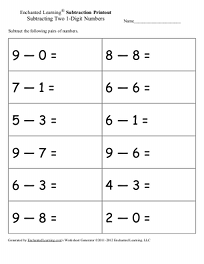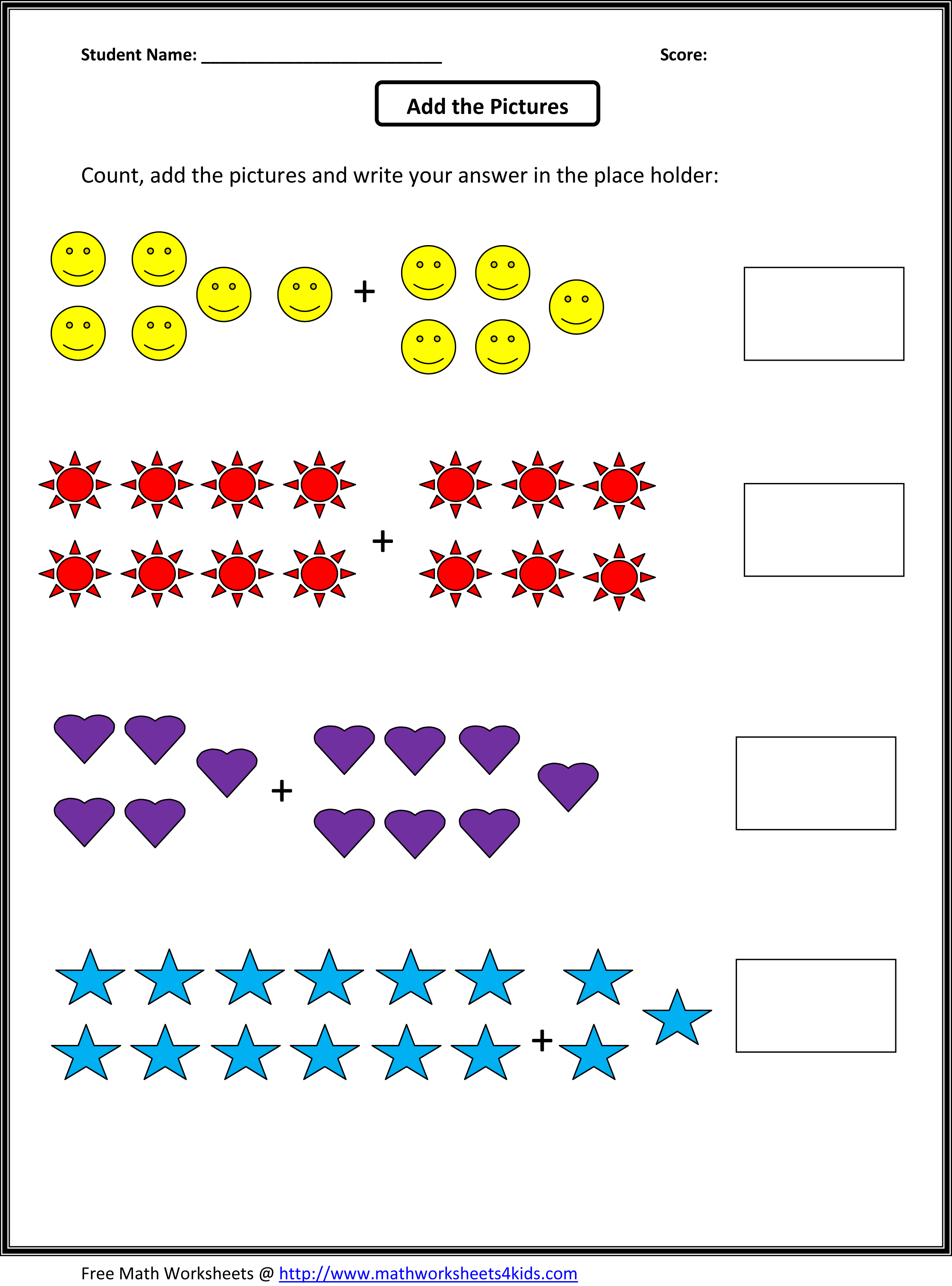# RH Penguin Homework

This week the Reception children learnt about the fantastic story ‘What’s in the Egg, Little Pip?’. The children learnt to write interesting facts about the penguins in Antarctica.

Watch the video below about the African Penguin to help you do the homework:

Literacy:

GreenBlue group: Edd and Eppa told us lots of interesting facts about the African penguin. Now make your own penguin fact book and write 4 different facts about the penguin.

If you are really clever can you use your research skills to find different places around the world where penguins can be found. Claim a prize for being a super explorer.

YellowOrange groups: Draw a picture of the African penguin and write 3 interesting facts about the penguin. Remember to use capital letters, finger spaces and full-stops in your sentences.

Red group: Draw a picture of the penguin and use your phonics knowledge to help you write some words to describe the penguin.

Maths:

GreenBlue group: Mr Harwood really needs your help to solve the sums below, especially as they are missing the addition and subtraction signs. Can you find which signs (+ or -) belong in the sums below to prove you are a super mathematician.YellowOrange groups: Write and solve the subtraction sums below. Take a look around your home and see if you can find 10 items. You might be able to find 10 pennies or 10 buttons. Use these as counters to help  you to solve the subtraction sums.Red Group: Write and solve the addition sum for each of the questions.A prize will be given to those who complete all of the homework.

## 14 thoughts on “RH Penguin Homework”

1. Rehan Hussain

9–0=9
8_8=0
7-1=6
6_6=0
5_3=2
6_1=5
9-7=2
6_3= 3
4_3=1
9_8=1
2_0=2

2. J Roberts

Well done Masoom you read the questions carefully and got them all correct. I wonder if you could write some more and bring them in for me to see.

3. Jawariyah Ashraf

Penguin its a bird but cant flay .
penguin always live by the sea
and they love eating fish the swimming very fast.

Maths
6+3=9
2+5=7
10_4=6
4+1=5
7_3=4
8_6=2
3+7=10
9_5=4
8+1=9
5_2=3

4. Lakeysha Mawela

Orange group
3 interesting facts about african penguins:
-African penguins feeds on small fish like sardines,pilchards and shellfish.
-African penguins are the fast swimmers and they can dive.
-They clean one other with their beaks.
Maths
Orange group
9-0=9
7-1=6
5-3=2
9-7=2
6-3=3
9-8=1
8-8=0
6-6=0
6-1=5
9-6=3
4-3=1
2-0=2

• J Roberts

Great work Lakeysha you listened carefully and found 3 facts about the penguins. Super work solving all the take away questions. Can you write some more and bring them in to show me?

5. Favour Nwanekwu

6+3=9
2+5=7
10-4=6
4+1=5
7-3=4
8-6=2
3+7=10
9-5=4
8+1=9
5-3=2
7-3=4

• J Roberts

Well done you read the number sentences carefully to see if it was a add or take away problem and got them all correct. Did you remember to make your Penguin fact book I’m looking forward to reading it.

6. Favour Nwanekwu

why don’t the penguin fly?

• J Roberts

Think carefully Victory we talked about penguins flying in class. Where do penguins fly? I’ll be asking you tomorrow.

7. Oliwier Janur

The penguins are white and black color.
May’s feet and short wings.
Love to eat fish and swim.

• J Roberts

Well done Oliwier you remembered capital letter, finger spaces and full stop when writing 3 facts about the Penguins.

8. Mujammil Ahmed

1. Penguin do not fly and do not use their wings other than to swim.
2. Penguin spend most of their life in or near the ocean.
3. The body of a penguin is covered short thick waterproof feathers.
4. Penguin clean each other with beaks.

Penguin can be found in New Zealand, The Galapagos Islands and north and south pole.

• Mujammil Ahmed

6+3=9

2+5=7
10-4=6
4+1=5
7-3=4
8-6=2
3+7=10
9-5=4
8+1=9
5-2=3
7-3=4

• J Roberts

Great facts about the penguins and super work finding out were the penguins live. I did not know they could be found in the Galapagos Islands, I have learnt something new as well. Thankyou